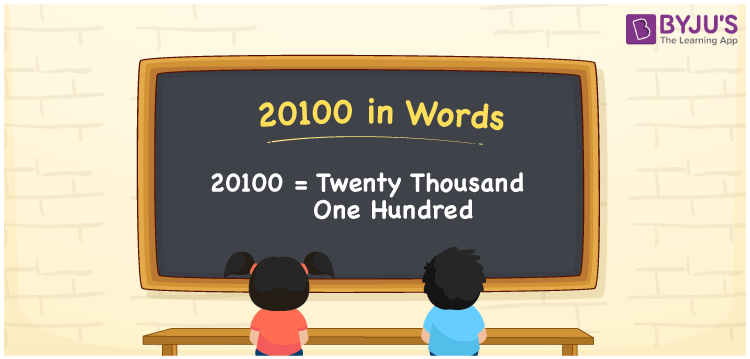# 20100 in words

20100 in words is written as Twenty Thousand One Hundred . In 20100, 2 has a place value of ten thousand, 9 is in the place value of hundred. The article on Place Value gives more information. The number 20100 is used in expressions that relate to money, distance, length, Social media view, and many more. For example, “The articles in a newspaper have Twenty Thousand One Hundred words on the front page.” Another example, “Rita’s Employee number is Twenty Thousand One Hundred.”

 20100 in words Twenty Thousand One Hundred Twenty Thousand One Hundred in Numbers 20100

## 20100 in English Words## How to Write 20100 in Words?

We can convert 20100 to words using a place value chart. The number 20100 has 5 digits, so let’s make a chart that shows the place value up to 5 digits.

 Ten thousand Thousands Hundreds Tens Ones 2 0 1 0 0

Thus, we can write the expanded form as:

2 × Ten thousand + 0 × Thousand + 1 × Hundred + 0 × Ten + 0 × One

= 2 × 10000 + 0 × 1000 + 1 × 100 + 0 × 10 + 0 × 1

= 20100.

= Twenty Thousand One Hundred.

20100 is the natural number that is succeeded by 20099 and preceded by 20101.

20100 in words – Twenty Thousand One Hundred.

Is 20100 an odd number? – No.

Is 20100 an even number? – Yes.

Is 20100 a perfect square number? – No.

Is 20100 a perfect cube number? – No.

Is 20100 a prime number? – No.

Is 20100 a composite number? – Yes.

## Solved Example

1. Write the number 20100 in expanded form

Solution: 2 x 10000 + 0 x 1000 + 1 x 100 + 0 x 10 + 0 x 1

Or Just 2 x 10000 + 1 x 100

We can write 20100 = 20000 + 0000 + 100 + 0 + 0

= 2 x 10000 + 0 x 1000 + 1 x 100 + 0 x 10 + 0 x 1.

## Frequently Asked Questions on 20100 in words

Q1

### How to write the number 20100 in words?

20100 in words is written as Twenty Thousand One Hundred.
Q2

### State whether True or False. 20100 is divisible by 3?

True. 20100 is divisible by 3.
Q3

### Is 20100 divisible by 10?

Yes. 20100 is divisible by 10.